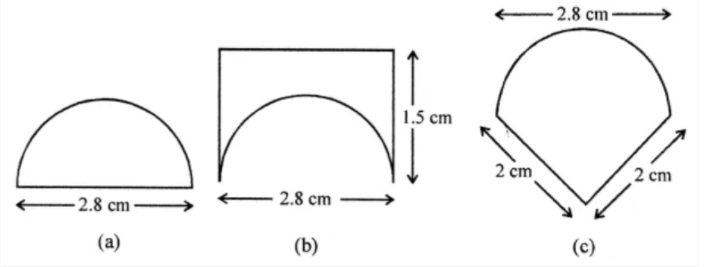# ML Aggarwal Solutions Class 8 Mathematics Solutions for Mensuration Exercise 18.1 in Chapter 18 - Mensuration

An ant is moving around a few food pieces of different shapes scattered on the floor. For which food piece would the ant have to take a longer round?(a) Diameter of semicircle = 2.8 cm

∴ Perimeter = πr + 2r

= 22/7 × 2.8 + 2 × 2.8

= 8.8 + 5.6 cm

= 14.4 cm

(b) Total perimeter = 1.5 + 1.5 + 2.8 + semi circumference (πr, where r = 2.8/2 = 1.4 cm)

= 1.5 + 1.5 + 2.8 + (22/7 x 1.4)

= 5.8 + 8.8

= 14.6 cm

(c) Total perimeter = 2 + 2 + Semi circumference (πr, where r = 2.8/2 = 1.4 cm)

= 4 + 8.8

= 12.8 cm

Hence, it is clearly seen that distance of (b) i.e. 14.6 is the longest.

Related Questions

Lido

Courses

Teachers

Book a Demo with us

Syllabus

Maths
CBSE
Maths
ICSE
Science
CBSE

Science
ICSE
English
CBSE
English
ICSE
Coding

Terms & Policies

Selina Question Bank

Maths
Physics
Biology

Allied Question Bank

Chemistry
Connect with us on social media!